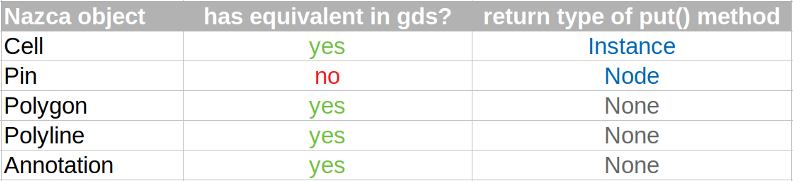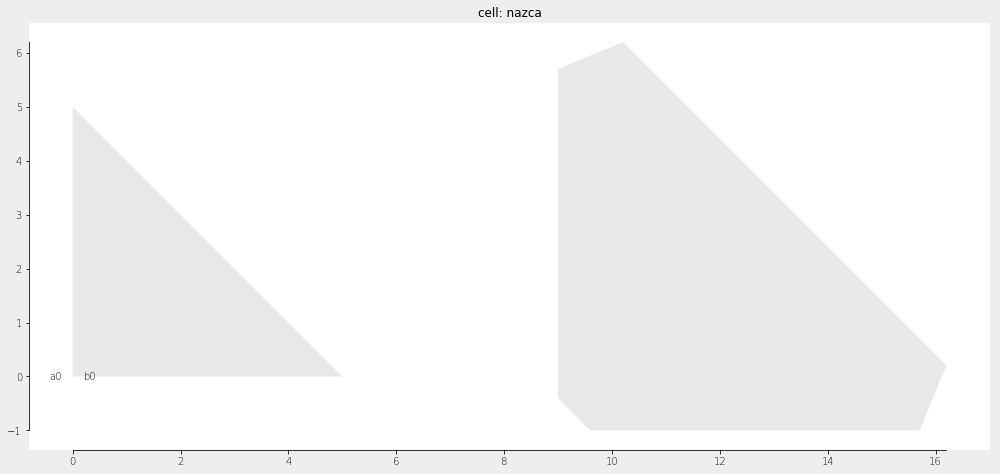Home Forums Nazca Subtracting two rectangle polygons

Viewing 3 posts - 1 through 3 (of 3 total)
• Author
Posts
• #5690

Hello,

I was wondering if I can get some advice on how to use the nazca.clipper.diff_polygons functions. Specifically on subtracting a small rectangle from a bigger rectangle. I’m new to python, nazca, and klayout so I might’ve just missed something easy when I searched on forums but I keep on getting TypeError that says “must be real number, not NoneType”

``````base = nd.Polygon(geom.parallelogram(length=7333.87, height=3803.27,
angle=90, position=1, shift=(0,0)), layer=2).put(0, 0)

straights = nd.Polygon(geom.parallelogram(length=6180.09, height=758,
angle=90, position=1, shift=(0,0)), layer=3).put(1153.78, 0)

nd.clipper.diff_polygons(base, straights, accuracy=0.001)``````

#5693

Dear Theflyingpengin,

When you put() an object, the return type is None, which explains the error message. You can easily check this by printing e.g. the type of base:

`print(type(base))`

The polygon operations of the clipper module require lists of polygons, where a polygon is a list of (x,y) values. So the first and second argument of diff_polygons() should be something like [[(1,2),(3,4),(5,1)]].

A small working example similar to your example (but with different polygon placement) would be:

```import nazca as nd
import nazca.geometries as geom

base = geom.parallelogram(length=7333.87, height=3803.27, angle=90, position=1, shift=(0, 0))
# base is a polygon and [base] is a list of polygons (with only one polygon).
straights = geom.parallelogram(length=6180.09, height=758, angle=90, position=1, shift=(0, 0))
polygons = nd.clipper.diff_polygons([base], [straights], accuracy=0.001)

for pol in polygons:  # There is only one polygon in the result in this case.
nd.Polygon(pol, layer=4).put()

nd.export_gds()```

Hope that helps you to go on.

Lastly a word of warning: subtracting or combining polygons may result in a “hole”. This is not handled by Nazca, because GDS cannot handle such polygons.

Xaveer

#5694

To add Xaveer’s answer, there are 5 class objects in Nazca with a put() method. Some of these objects have an equivalent in GDS and most of these put methods have return type None, including the Polygon. See the overview below.The Pyclipper needs to work on polygon points as Xaveer explained. For the ‘grow’ this has also been implemented as a Polygon class method, which internally operates on the polygon stored in the Polygon object.

``````import nazca as nd

pgon1 = nd.Polygon(points=[(0, 0), (5, 0), (0,5)])
pgon2 = pgon1.grow(grow=1)
pgon1.put(0)
pgon2.put(10)
nd.export_plt()``````Ronald

Viewing 3 posts - 1 through 3 (of 3 total)
• You must be logged in to reply to this topic.